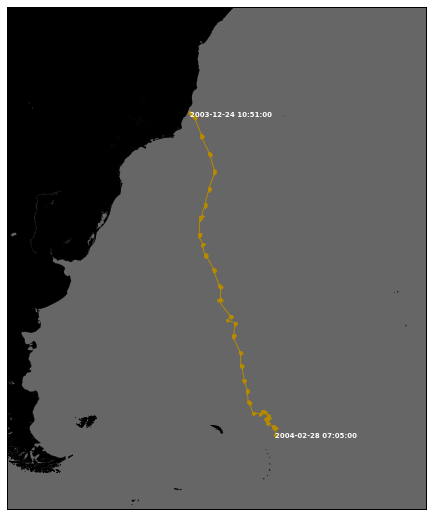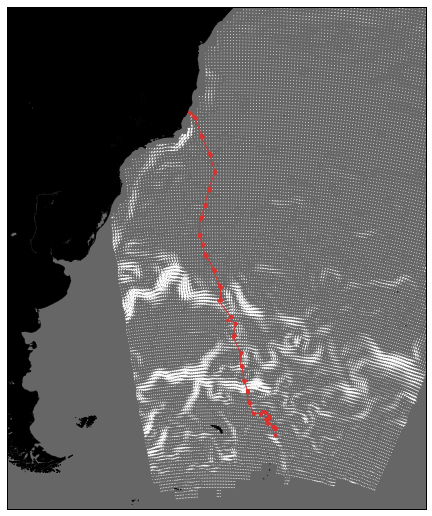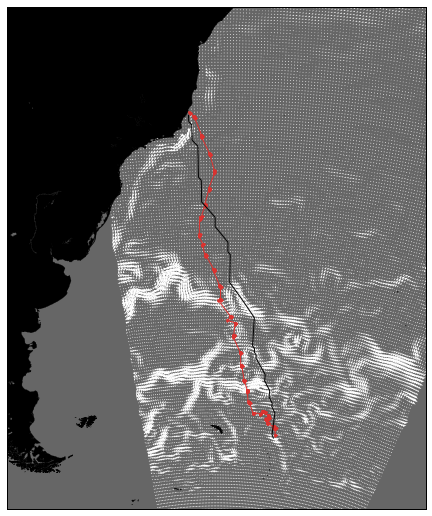# Analyzing whale tracks

Dr. Roberto De Almeida

Notebook file

In this iPython notebook we use ocean data to look at the trajectory of a migrating whale. When traveling on the surface of the Earth one cannot take a constant heading (an angle with respect to North) to travel the shortest route from point $A$ to $B$. Instead, the heading must be constantly readjusted so that the arc of the trajectory corresponds to the intersection between the globe and a plane that passes through the center of the Earth:This is called Great-circle Navigation, and is done by airplanes and ships (wherever possible).

There are also other factors that define the shortest route in time when travelling from $A$ to $B$. For airplanes the wind is an important factor (the jet streams are the reason why it's faster to fly from west to east compared to east to west), and for ships the ocean currents, tides and storms may be an important factor.

What about whales? I always wondered if whales could benefit from the ocean currents when migrating, by travelling along favorable currents and avoiding counter-currents. To investigate this, we develop here an algorithm for identifying the optimal path along two points considering a 2D field of ocean velocity that varies in time. We then compare the whale track with the optimal path to see how much they agree.

## The data¶

The data used corresponds to a track of a humpback whale travelling from the coast of Brazil to the Southern Ocean. The whale started its migration on 2003-12-24 leaving from 20.465 S, 40.04 W, and finished on 2004-02-28 at 54.67 S, 26.261 W:

In :
track = np.genfromtxt('traj.csv', delimiter=',', names=['time', 'lat', 'lon'], dtype=None,
converters={0: lambda s: datetime.datetime.strptime(s, '%m/%d/%Y %H:%M')})

print track[[0, -1]]

[(datetime.datetime(2003, 12, 24, 10, 51), -20.465, -40.04)
(datetime.datetime(2004, 2, 28, 7, 5), -54.67, -26.261)]


To plot the data we use the Basemap toolkit from Matplotlib:

In :
from mpl_toolkits.basemap import Basemap


And here is the trajectory of the whale:

In :
fig = figure(figsize=(15,9))
ax = fig.gca()

width = 5000000
height = 6000000
m = Basemap(width=width, height=height, projection='aeqd', lat_0=-36, lon_0=-37, resolution='h')
m.drawcoastlines(linewidth=0.25)
m.fillcontinents(color='#000000', lake_color='#666666')
m.drawmapboundary(fill_color='#666666')

color = "#b58900"
xx, yy = m(track['lon'], track['lat'])
m.plot(xx, yy, '-', color=color)
m.plot(xx, yy, '.', color=color)

plt.text(xx, yy, track['time'], fontsize=7, weight='bold', va='center', ha='left', color='w')
plt.text(xx[-1], yy[-1], track['time'][-1], fontsize=7, weight='bold', va='center', ha='left', color='w')For the ocean current data I've created a dataset in Marinexplore with OSCAR products from 2003-12-01 to 2004-03-01 for the South Atlantic. We can access it using Pydap as an Opendap client:

In :
# I'm using the development version from http://code.dealmeida.net/pydap
from pydap.client import open_url

print dataset




Here we are going to use the data only between 65 S and the equator, and from 50 W to 5 W:

In :
lon = dataset.lon0[:]
i0 = (lon <= -50).nonzero().max()
i1 = (lon < -5).nonzero().max() + 1
lon = lon[i0:i1]

lat = dataset.lat0[:]
j0 = (lat >= 0).nonzero().max()
j1 = (lat > -65).nonzero().max() + 1
lat = lat[j0:j1]

U = dataset.g_current_speed_vector_u.g_current_speed_vector_u[:,:,j0:j1,i0:i1]
V = dataset.g_current_speed_vector_v.g_current_speed_vector_v[:,:,j0:j1,i0:i1]

# remove vertical dimension and mask NaN
U = U[:,0,:,:]
U[isnan(U)] = 0.0
V = V[:,0,:,:]
V[isnan(V)] = 0.0

import coards
T = np.array([coards.format(coards.parse(value, dataset.time0.units), 'seconds since 1970-01-01') for value in dataset.time0])


And here is the average ocean current field during the period:

In :
fig = figure(figsize=(15,9))
ax = fig.gca()

width = 5000000
height = 6000000
m = Basemap(width=width, height=height, projection='aeqd', lat_0=-36, lon_0=-37, resolution='h')
m.drawcoastlines(linewidth=0.25)
m.fillcontinents(color='#000000', lake_color='#666666')
m.drawmapboundary(fill_color='#666666')

X, Y = np.meshgrid(lon, lat)
xx, yy = m(X, Y)
m.quiver(xx, yy, np.mean(U, axis=0), np.mean(V, axis=0), color='white')

color = "#dc322f"
xx, yy = m(track['lon'], track['lat'])
m.plot(xx, yy, '-', color=color)
m.plot(xx, yy, '.', color=color)

Out:
[]In order to calculate the shortest path between the starting and finishing positions of the whale we need a few accessory functions. First, we need to be able to calculate the distance between two points in the sphere. While on a flat surface we could use Pythagora's theorem, on a sphere the calculations are bit more complicated:

In :
def distance(lat1, lon1, lat2, lon2):
# http://www.movable-type.co.uk/scripts/latlong.html
R = 6.371e6
lat1 *= pi/180.
lon1 *= pi/180.
lat2 *= pi/180.
lon2 *= pi/180.
return R*np.arccos(
np.sin(lat1)*np.sin(lat2) +
np.cos(lat1)*np.cos(lat2)*np.cos(lon2-lon1))


We also need to calculate the heading between two points. This is important because given two points we need to calculate the speed of the current along the path between them, and this is done by projecting the $u$ and $v$ components of the ocean current along the path:

$s = s0 + u\cos(a) + v\sin(a)$

Where $s$, the resulting speed along a path, is given by the whale velocity $s0$, plus the projection of the currents on the path with heading $a$. The formula to calculate the heading between two points on the sphere is:

In :
def bearing(lat1, lon1, lat2, lon2):
# http://www.movable-type.co.uk/scripts/latlong.html
lat1 *= pi/180.
lon1 *= pi/180.
lat2 *= pi/180.
lon2 *= pi/180.
y = np.sin(lon2-lon1)*np.cos(lat2)
x = np.cos(lat1)*np.sin(lat2) - np.sin(lat1)*np.cos(lat2)*np.cos(lon2-lon1)
return (pi/2) - np.arctan2(y, x)


We now adapt Dijkstra's algorithm to determine the optimal route. Since OSCAR is gridded, we'll use the grid points as the nodes of our graph, connecting vertical, horizontal and diagonal grid points to form the graph. The cost to travel between two nodes is determine by the time to travel from one to another, and depends on the distance between them and the current field, which will vary in time.

First let's construct our graph, by connecting neighbors:

In :
x = lon
y = lat

G = {}
for i in range(len(x)):
for j in range(len(y)):
G[j, i] = []
if i > 0:
if j > 0:
G[j, i].append((j-1, i-1))
if j+1 < len(y):
G[j, i].append((j+1, i-1))
G[j, i].append((j, i-1))
if i+1 < len(x):
if j > 0:
G[j, i].append((j-1, i+1))
if j+1 < len(y):
G[j, i].append((j+1, i+1))
G[j, i].append((j, i+1))
if j > 0:
G[j, i].append((j-1, i))
if j+1 < len(y):
G[j, i].append((j+1, i))


In our graph G the node (j, i) corresponds to the grid cell at indexes (j, i) from the OSCAR dataset.

We then implement Dijkstra's algorithm with one simple change: the travel cost between two nodes will depend on the time when the node is reached, since the currents are non-stationary. This means that nodes maybe be revisited, since they may be reached at different times from different paths, resulting in different costs:

In :
import heapq

def shortest_path(X, Y, U, V, T, G, start, end, s0=0, t0=0):
q = [(t0, start, ())]
visited = {}
while 1:
cost, v1, path = heapq.heappop(q)
if v1 not in visited or visited[v1] > cost:
path = path + (v1,)
visited[v1] = cost
if v1 == end:
return list(path), cost
for v2 in G[v1]:
cost2 = calculate_cost(X, Y, U, V, T, cost, v1, v2, s0)
if (v2 not in visited or visited[v2] > cost2) and cost+cost2 <= T[-1]:
heapq.heappush(q, (cost + cost2, v2, path))


In the function X and Y are 2D dimensional arrays representing the coordinates, U and V 3D arrays representing the time-varying ocean currents, T is a vector with time values, G is our graph, start and time are nodes in the graph, s0 is the whale mean speed and t0 is the starting time.

We now need to define our calculate_cost function, which returns the time to travel between nodes v1 and v2 at a given instant in time; note that in the algorithm above the current time is given by the variable cost, which represents the current total cost of the path.

In :
def calculate_cost(X, Y, U, V, T, t, v1, v2, s0):
l = (T <= t).nonzero().max()
j1, i1 = v1
j2, i2 = v2

u = (U[l,j1,i1] + U[l,j2,i2])/2.
v = (V[l,j1,i1] + V[l,j2,i2])/2.

ds = distance(Y[v1], X[v1], Y[v2], X[v2])
a = bearing(Y[v1], X[v1], Y[v2], X[v2])

# velocity along track
s = s0 + u*np.cos(a) + v*np.sin(a)
if s < 0:
return np.inf
else:
return ds/s


All we need to do now is to determine the inital and finishing nodes, based on the whale track:

In :
# get initial and final nodes
i = (x <= track['lon']).nonzero().max()
j = (y <= track['lat']).nonzero().min()
v1 = j, i
i = (x <= track['lon'][-1]).nonzero().max()
j = (y <= track['lat'][-1]).nonzero().min()
v2 = j, i

s0 = 0.86
t0 = coards.format(track['time'], 'seconds since 1970-01-01')
path, end = shortest_path(X, Y, U, V, T, G, v1, v2, s0, t0)


Here is the optimal trajectory, compared to the whale track:

In :
fig = figure(figsize=(15,9))
ax = fig.gca()

width = 5000000
height = 6000000
m = Basemap(width=width, height=height, projection='aeqd', lat_0=-36, lon_0=-37, resolution='h')
m.drawcoastlines(linewidth=0.25)
m.fillcontinents(color='#000000', lake_color='#666666')
m.drawmapboundary(fill_color='#666666')

X, Y = np.meshgrid(lon, lat)
xx, yy = m(X, Y)
m.quiver(xx, yy, np.mean(U, axis=0), np.mean(V, axis=0), color='white')

color = "#dc322f"
xx, yy = m(track['lon'], track['lat'])
m.plot(xx, yy, '-', color=color)
m.plot(xx, yy, '.', color=color)

xx = [X[s] for s in path]
yy = [Y[s] for s in path]
xx, yy = m(xx, yy)
m.plot(xx, yy, 'k-')

Out:
[]We can see that both the optimal (black) and the whale (red) trajectory seem to follow current patters in the Brazil-Falklands confluence, and that the trajectories are similar. In order to properly evaluate if whales indeed benefit from the ocean currents more data is needed, but the results and the methodology seem very promising.